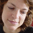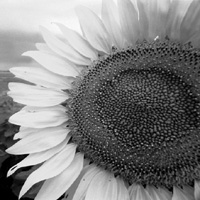# Processing.js

## Convolution

by Daniel Shiffman. Applys a convolution matrix to a portion of the index. Move mouse to apply filter to different parts of the image.

Original Processing.org Example: Convolution```// All Examples Written by Casey Reas and Ben Fry
// unless otherwise stated.
PImage img;
int w = 80;

// It's possible to convolve the image with
// many different matrices

float[][] matrix = { { -1, -1, -1 },
{ -1,  9, -1 },
{ -1, -1, -1 } };

void setup() {
size(200, 200);
frameRate(30);
}

void draw() {
// We're only going to process a portion of the image
// so let's set the whole image as the background first
image(img,0,0);
// Where is the small rectangle we will process
int xstart = constrain(mouseX-w/2,0,img.width);
int ystart = constrain(mouseY-w/2,0,img.height);
int xend = constrain(mouseX+w/2,0,img.width);
int yend = constrain(mouseY+w/2,0,img.height);
int matrixsize = 3;
// Begin our loop for every pixel
for (int x = xstart; x < xend; x++) {
for (int y = ystart; y < yend; y++ ) {
color c = convolution(x,y,matrix,matrixsize,img);
int loc = x + y*img.width;
pixels[loc] = c;
}
}
updatePixels();
}

color convolution(int x, int y, float[][] matrix,int matrixsize, PImage img)
{
float rtotal = 0.0;
float gtotal = 0.0;
float btotal = 0.0;
int offset = matrixsize / 2;
for (int i = 0; i < matrixsize; i++){
for (int j= 0; j < matrixsize; j++){
// What pixel are we testing
int xloc = x+i-offset;
int yloc = y+j-offset;
int loc = xloc + img.width*yloc;
// Make sure we haven't walked off our image, we could do better here
loc = constrain(loc,0,img.pixels.length-1);
// Calculate the convolution
rtotal += (red(img.pixels[loc]) * matrix[i][j]);
gtotal += (green(img.pixels[loc]) * matrix[i][j]);
btotal += (blue(img.pixels[loc]) * matrix[i][j]);
}
}
// Make sure RGB is within range
rtotal = constrain(rtotal,0,255);
gtotal = constrain(gtotal,0,255);
btotal = constrain(btotal,0,255);
// Return the resulting color
return color(rtotal,gtotal,btotal);
}```